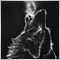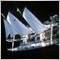# Take profit candle pips576

Hi everyone, I'm a beginner and I'm trying to learn. I have a problem, in this practice expert, I insert the stop loss exactly on the minimum of the candle and I would like the RR ratio to be 1 to 1. I'm trying to calculate the difference in pips between the maximum and the minimum and then add it to my entry price, but it includes a take profit too close.

```//+------------------------------------------------------------------+
//|                                              engulfingh4test.mq4 |
//|                        Copyright 2018, MetaQuotes Software Corp. |
//|                                             https://www.mql5.com |
//+------------------------------------------------------------------+
#property version   "1.00"
#property strict
extern double    Lots=0.1;
double stoploss = NormalizeDouble((1000*Point),Digits);
double takeprofitsell = MathAbs(NormalizeDouble(iHigh(NULL,0,1)+iLow(NULL,0,1),5)*Point);
datetime NewTime=0;
//+------------------------------------------------------------------+
//| Expert initialization function                                   |
//+------------------------------------------------------------------+
int OnInit()
{
//---

//---
return(INIT_SUCCEEDED);
}
//+------------------------------------------------------------------+
//| Expert deinitialization function                                 |
//+------------------------------------------------------------------+
void OnDeinit(const int reason)
{
//---

}
//+------------------------------------------------------------------+
//| Expert tick function                                             |
//+------------------------------------------------------------------+
void OnTick()
{
if(NewTime!=Time) // Run only on new bars
{
NewTime=Time;
double media = iMA(Symbol(),0,30,0,MODE_EMA,PRICE_CLOSE,1);
bool orderOpended=false;
iOpen(NULL,0,2)>iClose(NULL,0,2) && // Place Buy logic in if statemet

else if(Bid<media &&
iOpen(NULL,0,2)<iClose(NULL,0,2) && // Place Sell logic in if satement
iHigh(NULL,0,1)>iHigh(NULL,0,2) &&       // Sell logic
iLow(NULL,0,1)<iLow(NULL,0,2))         // Sell logic
orderOpended=OrderSend(Symbol(),OP_SELL,Lots,Bid,3,iHigh(NULL,0,1),Bid-takeprofitsell,NULL,0,0,Red);
}
}
//+------------------------------------------------------------------+
```533

To get the Candle "pips" you should be subtracting the "Low" from the "High" of the candle surely.... Also, you need to be dividing that value with Point (not multiplying)... that is why you are getting such a small take profit value...

`double takeprofitbuy = MathAbs(NormalizeDouble(iHigh(NULL,0,1)-iLow(NULL,0,1),5)/Point);`

You also only need one "takeprofit" variable... as you will always be subtracting the Low from the High...

Hope that works and I am understanding your question correctly...576

Mike Tanton :

To get the Candle "pips" you should be subtracting the "Low" from the "High" of the candle surely.... Also, you need to be dividing that value with Point (not multiplying)... that is why you are getting such a small take profit value ...

You also only need one "takeprofit" variable... as you will always be subtracting the Low from the High...

Hope that works and I am understanding your question correctly...

I tried, but it does not work. Take profit becomes an absurd value. Strangling, removing * point and digits, seems to work properly (although sometimes the value of sl seems to me less than the tp)

```//+------------------------------------------------------------------+
//|                                              engulfingh4test.mq4 |
//|                        Copyright 2018, MetaQuotes Software Corp. |
//|                                             https://www.mql5.com |
//+------------------------------------------------------------------+
#property version   "1.00"
#property strict
extern double    Lots=0.1;
double stoploss = NormalizeDouble((1000*Point),Digits);
double takeprofit = MathAbs(iHigh(Symbol(),0,1)-iLow(Symbol(),0,1));
datetime NewTime=0;
//+------------------------------------------------------------------+
//| Expert initialization function                                   |
//+------------------------------------------------------------------+
int OnInit()
{
//---

//---
return(INIT_SUCCEEDED);
}
//+------------------------------------------------------------------+
//| Expert deinitialization function                                 |
//+------------------------------------------------------------------+
void OnDeinit(const int reason)
{
//---

}
//+------------------------------------------------------------------+
//| Expert tick function                                             |
//+------------------------------------------------------------------+
void OnTick()
{
if(NewTime!=Time) // Run only on new bars
{
NewTime=Time;
double media = iMA(Symbol(),0,30,0,MODE_EMA,PRICE_CLOSE,1);
bool orderOpended=false;
iOpen(NULL,0,2)>iClose(NULL,0,2) && // Place Buy logic in if statemet

else if(Bid<media &&
iOpen(NULL,0,2)<iClose(NULL,0,2) && // Place Sell logic in if satement
iHigh(NULL,0,1)>iHigh(NULL,0,2) &&       // Sell logic
iLow(NULL,0,1)<iLow(NULL,0,2))         // Sell logic
orderOpended=OrderSend(Symbol(),OP_SELL,Lots,Bid,3,iHigh(NULL,0,1),Bid-takeprofit,NULL,0,0,Red);
}
}
//+------------------------------------------------------------------+
```533

Sorry... yes the "takeprofit" value shoud be multiplied by Point as you originally had it... so, you need to put that back in...

`double takeprofit = MathAbs(iHigh(Symbol(),0,1)-iLow(Symbol(),0,1)) * Point;`

...that will then give you the correct "takeprofit" point size.... so your Take Profit levels should now work ok...576

Roberto Danilo:

I tried, but it does not work. Take profit becomes an absurd value. Strangling, removing * point and digits, seems to work properly (although sometimes the value of sl seems to me less than the tp)

no, the strange thing is that no point works ... it is with the point that gives me the take profit at the same price of the entry level ... I do not know why533

I am confusing you Roberto.... There should not be any "Point" in the calculation because the "High" minus the "Low" gives you the difference in "Points".... which is what you need as your "takeprofit" value...

`double takeprofit = MathAbs(iHigh(Symbol(),0,1)-iLow(Symbol(),0,1));`576

Mike Tanton :

I am confusing you Roberto.... There should not be any "Point" in the calculation because the "High" minus the "Low" gives you the difference in "Points".... which is what you need as your "takeprofit" value...

We would miss Mike, you are very kind to help me. Now I would like to set the breakeven function when the price comes in half from the take profit ... I entered the function, but does not seem to go, no operation is closed in breakeven. Maybe I'm wrong method to get half of my take profit?

```//+------------------------------------------------------------------+
//|                                              engulfingh4test.mq4 |
//|                        Copyright 2018, MetaQuotes Software Corp. |
//|                                             https://www.mql5.com |
//+------------------------------------------------------------------+
#property version   "1.00"
#property strict
extern double    Lots=0.1;
double stoploss = NormalizeDouble((1000*Point),Digits);
double takeprofit = MathAbs(iHigh(Symbol(),0,1)-iLow(Symbol(),0,1));
datetime NewTime=0;
double    Breakeven = MathAbs(takeprofit/2);
input double    Magic = 280456;
//+------------------------------------------------------------------+
//| Expert initialization function                                   |
//+------------------------------------------------------------------+
int OnInit()
{
//---

//---
return(INIT_SUCCEEDED);
}
//+------------------------------------------------------------------+
//| Expert deinitialization function                                 |
//+------------------------------------------------------------------+
void OnDeinit(const int reason)
{
//---

}
//+------------------------------------------------------------------+
//| Expert tick function                                             |
//+------------------------------------------------------------------+
void OnTick()
{
if(NewTime!=Time)
{
NewTime=Time;
double media = iMA(Symbol(),0,30,0,MODE_EMA,PRICE_CLOSE,1);
bool orderOpended=false;
iOpen(NULL,0,2)>iClose(NULL,0,2) && // Place Buy logic in if statemet

else if(Bid<media &&
iOpen(NULL,0,2)<iClose(NULL,0,2) && // Place Sell logic in if satement
iHigh(NULL,0,1)>iHigh(NULL,0,2) &&       // Sell logic
iLow(NULL,0,1)<iLow(NULL,0,2))         // Sell logic
orderOpended=OrderSend(Symbol(),OP_SELL,Lots,Bid,3,Bid+takeprofit,Bid-takeprofit,NULL,Magic,0,Red);
}

}
//+------------------------------------------------------------------+
void BreakEven()
{
double MyPoint=Point;
if(Digits==3 || Digits==5) MyPoint=Point*10;

for(int i=OrdersTotal()-1; i>=0;i--)
{
if(OrderSymbol()==Symbol())
if(OrderMagicNumber()==Magic)
{
if(Bid-OrderOpenPrice()>Breakeven*MyPoint)
if(OrderOpenPrice()>OrderStopLoss())
int BBM=OrderModify(OrderTicket(),OrderOpenPrice(),OrderOpenPrice(),OrderTakeProfit(),0,clrNONE);
}

else if(OrderType()==OP_SELL)
{
if(OrderOpenPrice()<OrderStopLoss())
int BSM=OrderModify(OrderTicket(),OrderOpenPrice(),OrderOpenPrice(),OrderTakeProfit(),0,clrNONE);
}
}
}

```533

Hi Roberto... why have you forgotten about your "takeprofit" and Breakeven" variables...

```// For Long positions
int BBM=OrderModify(OrderTicket(),OrderOpenPrice(),OrderOpenPrice()+Breakeven,OrderOpenPrice()+takeprofit,0,clrNONE);

// For Short positions
int BBM=OrderModify(OrderTicket(),OrderOpenPrice(),OrderOpenPrice()-Breakeven,OrderOpenPrice()-takeprofit,0,clrNONE);```

Also,... it looks like you are still having issues with calculating the Point value of your variables... and your BreakEven() function is a bit of a mess... Try this...

```//+-------------------------+
//| Break Even Function     |
//+-------------------------+
void BreakEven()
{
double MyPoint=Point;
if(Digits==3 || Digits==5) MyPoint=Point*10;

for(int i=OrdersTotal()-1; i>=0;i--)
{
&& OrderSymbol()==Symbol()
&& OrderMagicNumber()==Magic)
{
{
int BBM=OrderModify(OrderTicket(),OrderOpenPrice(),OrderOpenPrice()+Breakeven,OrderOpenPrice()+takeprofit,0,clrNONE);
}

if(   OrderType()==OP_SELL
&& OrderOpenPrice()-Bid>Breakeven)
{
int BBM=OrderModify(OrderTicket(),OrderOpenPrice(),OrderOpenPrice()-Breakeven,OrderOpenPrice()-takeprofit,0,clrNONE);
}
}
}
}```

See if that works....

Don't forget to "Call" the Breakeven function....

```//+------------------------------------------------------------------+
//| Expert tick function                                             |
//+------------------------------------------------------------------+
void OnTick()
{
BreakEven();```576

Mike Tanton :

Hi Roberto... why have you forgotten about your "takeprofit" and Breakeven" variables...

Also,... it looks like you are still having issues with calculating the Point value of your variables... and your BreakEven() function is a bit of a mess... Try this...

See if that works....

Don't forget to "Call" the Breakeven function....

Thanks Mike for helping me. I forgot to put the fuction in OnTick, and now everything works fine.533

Sure... No Problem....good luck... go for it... and don't be afraid to try things out

If I can give you some advice before you start your next MT4/MT5 project.... spend some time reading up about "How to Structure EA's" and anything on "Good Coding Practice".... there is lots of good stuff out there... As your code becomes longer and more complicated the more important it is to have it all organised. If you can get into good habits NOW... then you will save yourself a whole lot of time and heartache later on....576

Mike Tanton :

Sure... No Problem....good luck... go for it... and don't be afraid to try things out

If I can give you some advice before you start your next MT4/MT5 project.... spend some time reading up about "How to Structure EA's" and anything on "Good Coding Practice".... there is lots of good stuff out there... As your code becomes longer and more complicated the more important it is to have it all organised. If you can get into good habits NOW... then you will save yourself a whole lot of time and heartache later on....